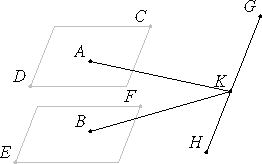# Proposition 14

Planes at right angles to the same straight line are parallel.

Let any straight line AB be at right angles to each of the planes CD and EF.

I say that the planes are parallel.For, if not, then they meet when produced. Let them meet. Then they intersect as a straight line. Let it be GH.

Take a point K at random on GH, and join AK and BK.

XI.Def.3

Now, since AB is at right angles to the plane EF, therefore AB is also at right angle to BK which is a straight line in the plane EF produced. Therefore the angle ABK is right. For the same reason the angle BAK is also right.

I.17

Thus, in the triangle ABK the sum of the two angles ABK and BAK equals two right angles, which is impossible.

XI.Def.8

Therefore the planes CD and EF do not meet when produced. Therefore the planes CD and EF are parallel.

Therefore, planes at right angles to the same straight line are parallel.

Q.E.D.

## Guide

Part of this proof is unnecessary. The line GH is irrelevant. If the two points meet, then they meet at some point, so the point K might just as well be taken as a point where they meet. The proof should, however, include another case, and that is where the given line meets both given planes at a point common to both planes.

#### Use of this proposition

This proposition is used in the proof of the next one.